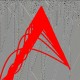## MOON4 - Moon Safari (Extreme)

no tags

This problem is a harder version of MOON2.

Your task is: given $N$, $a$ and $r$, compute

$$S(N, a, r) = \sum_{i=1}^N a^i i^r.$$

### Input

The first line contains an integer $T$, the number of test cases.
On the next $T$ lines, you will be given three integers $N$, $a$ and $r$.

### Output

Output $T$ lines, one for each test case, with $S(N, a, r)$.
Since the answer can get very big, output it modulo $10^9+7$.

### Example

Input:
23 4 56 7 8

Output:
16068329990641

### Constraints

Overall constraints:

• $1 \leq T \leq 10^5$
• $1 \leq N \leq 10^{18}$
• $1 \leq a \leq 10^{18}$
• $1 \leq r \leq 10^8$
•

More precise information: there are 6 test cases.

Test #0: $1 \leq T \leq 100000$ and $1 \leq r \leq 1000$.

Test #1: $1 \leq T \leq 10000$ and $1 \leq r \leq 10000$.

Test #2: $1 \leq T \leq 1000$ and $1 \leq r \leq 100000$.

Test #3: $1 \leq T \leq 100$ and $1 \leq r \leq 1000000$.

Test #4: $1 \leq T \leq 10$ and $1 \leq r \leq 10000000$.

Test #5: $T = 1$ and $1 \leq r \leq 100000000$.

### Information

Four trips on the moon are provided, Moon (easy), Moon1 (medium), Moon2 (hard), Moon4 (extreme) with different constraints.

Please pay attention to the constraints which may differ from the previous versions.

Also please handle the constraints carefully.

We do not provide the intended time complexity in order to encourage possible various ways of thinking.

My fastest C++ code got AC under in 12.14s. (approx 2.02s per file)

Good luck and have fun :-)

You may be surprised why the code of this problem is not Moon3. It is because

• 4 is a lucky number on the moon.
• This problem is the 4th one in the Moon series.
• 4 is a power of 2, which indicates exponential increasing difficulty starting from 2.
• Moon3 has been used.Francky: 2020-06-10 12:27:11 Done ;-) Maybe I'll find a way to set MOON3 as a new interesting trip, as I am the owner of MOON3 ; unreleased.zfwang: 2019-03-30 08:14:47 which has been used the name of Moon3 or the method of Moon3 =(liouzhou_101)=> Just a joke. It's the case that MOON3 has been used. Last edit: 2019-03-30 12:56:12Francky: 2019-03-14 18:08:24 This is Extreme. Thanks for this new trip !